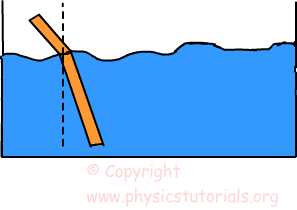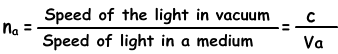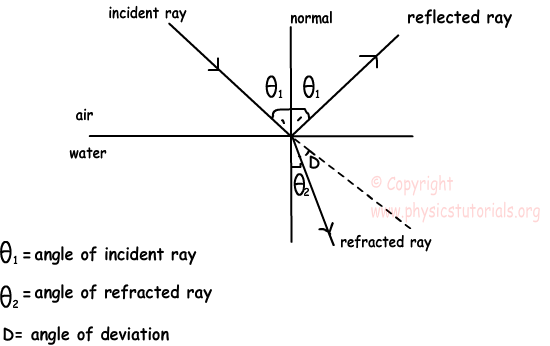## Refraction Of Light

Refraction

As I said before light travels in a straight line in any homogenous medium. However, when it passes from one medium to another medium itchanges its direction. We call this change in the direction of light refraction. Since the densities of the mediums are different light travels with different speed in different mediums. Speed of light in vacuum is 300.000.000km per hour. You see the stick as it is broken in the given picture. It is the clear example of refraction of light. We find the amount of refraction by using the refractive indexes of the mediums. What is the refractive index? It is the ratio of the speed of light in vacuum to the speed of the light in given medium. Refractive index of medium A is given below;Now we examine the refraction of light from two mediums. We assume the refractive index of air 1.The drawing given above shows the angles of reflected and refracted rays. You may some confusion in your mind about the reflection of the light in refracting. However, I want to mention it here, when an incident ray passes one medium to another some parts of it is reflected from the surface of boundary and the rest is refracted. The amount of reflection is related to the difference between the refractive indexes of the mediums. Later in this topic we will see this detail.

Optics Exams and Solutions

Author: